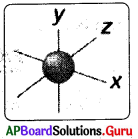Practice the AP 10th Class Physical Science Bits with Answers Chapter 6 Structure of Atom on a regular basis so that you can attempt exams with utmost confidence.

## AP State Syllabus 10th Class Physical Science Bits 6th Lesson Structure of Atom with Answers

CONCEPT -I: Electromagnetic ctrum

Question 1.
Match the following:
a) Electron — ii) Negatively charged particle
c) Neutron — iii) Neutral particle
A) a → i, b → ii, c → iii
B) c → i, b → ii, a → iii
C) a → ii, b → i, c → iii
D) a → ii, b → iii, c → i
C) a → ii, b → i, c → iiiQuestion 2.
The sub atomic particles present in an atom……………
i) electron
ii) proton
iii) neutron
A) only i
B) only ii
C) both i and ii
D) all of these
D) all of these

Question 3.
The number of colours in rainbow
A) 3
B) 5
C) 7
D) 9
C) 7

Question 4.
A vibrating charge produces ………………..
A) MechMhical wave
B) Stationary wave
C) Longitudinal wave
D) Electromagnetic wave
D) Electromagnetic wave

Question 5.
Electromagnetic wave travels with a speed of …………….
A) 3 x 107 m/s
B) 3 x 106 m/s
C) 3 x 108 m/s
D) 3 x 109 m/s
C) 3 x 108 m/s

Question 6.
Electromagnetic energy is charecterized by………………
i) wavelength
ii) amplitude
iii) frequency
A) only i
B) only ii
C) both i and iii
D) all of these
C) both i and iii

Question 7.
The distance between two successive peaks of a wave is …………….
A) Amplitude
B) Frequency
C) Wavelength
D) Speed
C) Wavelength

Question 8.
The relation between frequency and wavelength of electromagnetic wave
A) λ ∝ υ
B) λ = υ
C) λ ∝ 1/υ
D) none of these
C) λ ∝ 1/υ

Question 9.
Formation of rainbow is example of …………….. spectrum.
A) UV
B) IR
D) Visible
D) VisibleQuestion 10.
V = υλ, is applicable for……………..
A) longitudinal waves
B) transverse waves
C) electromagnetic waves
D) all
D) all

Question 11.
Red has …………………. wavelength and …………………. frequency.
A) maximum, maximum
B) minimum, maximum
C) maximum, minimum
D) minimum, minimum
C) maximum, minimum

Question 12.
Quantum theory was proposed by……………….
A) Einsteiry
B) Maxwell
C) Max Planck
D) Newton
C) Max Planck

Question 13.
Planck’s equation is …………..
A) E = hυ
B) E = h/υ
C) E = υ/h
D) None of these
A) E = hυ

Question 14.
Which of the following are correct statements:
P: E = ko is called Planck’s equation
Q: ‘h’ is called Planck’s constant
R: ‘h’ value is 6.626 x 10-34J
S: According to Planck electro-magnetic energy is always emitted in multiples of h/υ
A) onlyP
B) PandQ
C) P, Q and R
D) All of these
C) P, Q and R

Question 15.
When an iron rod is heated first it turns to
A) violet
B) red
C) green
D) orange
B) red

Question 16.
Cupric chloride produces ………….. colour flame.
A) red
B) green
C) blue
D) orange
B) green

Question 17.
Strontium chloride produces……………… colour flame.
A) red
B) green
C) blue
D) orange
A) red

Question 18.
…………….. vapours produce yellow light in street lamps.
A) Potassium
B) Sodium
C) Calcium
D) Magnesium
B) Sodium

Question 19.
Elements emit characteristic colours correspond to certain discrete wave¬lengths of light are called ……………..
A) bond spectra
B) absorption spectra
C) line spectra
D) both A and B
C) line spectra

Question 20.
Line spectra is formed by ………………
A) atoms
B) molecules
C) ions
D) protons
A) atoms

Question 21.
From the figure, the wavelength of Cosmic rays is………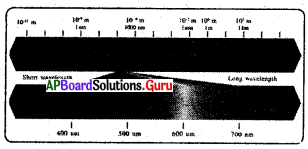A) 10-3 m
B) 10-9 m
C) 103 m
D) 10-12 m
D) 10-12 mQuestion 22.
The wavelength range of. visible spectrum is……….
A) <400 nm B) >700 nm
C) 400 nm – 700 nm
D) 1mm-lm
C) 400 nm – 700 nm

Question 23.
In this figure magnetic field and electric field are………. each other.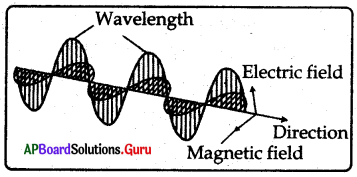A) parallel to
B) perpendicular to
C) intersect
D) None of these
B) perpendicular to

Question 24.
From the figure match the following:
a) X-rays — i) 700nm-1000nm
b) IRrays — ii) lm to 1 km
c) Radio rays — iii) Above 1 km
d) Broadcast — iv) 0.01 to 10 nm bond
A) a → iv, b → i, c → ii, d → iii
B) a →i, b → ii, c → iii, d → iv
C) a → iv, b → iii, c → ii, d → n
D) a → iii, b → iv, c →i, d → ii
A) a → iv, b → i, c → ii, d → iii

Question 25.
A) UVrays
B) IRrays
C) Microwaves
D) X-rays
C) Microwaves

Question 26.
An emission spectrum consists of , bright spectral lines on a dark back ground. Which one of the following does not correspond to the bright j spectral lines ?
D) Velocity of light
D) Velocity of light

Question 27.
The colour in the sequence VIBGYOR that has least wavelength is …………….
A) yellow
B) red
C) green
D) violet
D) violet

Question 28.
Which electromagnetic waves are sensitive to our eyes ?
A) X – rays
B) Ultraviolet rays
C) Visible rays
D) Microwaves
C) Visible rays

Question 29.
Rainbow is a …………….. spectrum.
A) continuous
B) discontinuous
C) visible
D) both A and C
D) both A and C

CONCEPT – II :Atomic model Bohr’s model & Sommer feld’s model

Question 30.
Stationary orbits are proposed by
A) Rutherford
B) Thomson
C) Newton
D) Neils Bohr
D) Neils Bohr

Question 31.
The energies of an electron in an orbit of atom can have only certain values. These orbits are called……………..
i) stationary orbits
ii) stationary states
iii) energy level,
A) only i
B) only ii
C) only iii
D) all of these
D) all of these

Question 32.
The energy is quantized means ………………
A) Energy is fixed
B) Energy would increase
C) Energy would decrease
D) None of these
A) Energy is fixedQuestion 33.
Lowest energy state of an electron is known as ……..
A) excited states
B) ground state
C) fixed state
D) rest state
B) ground state

Question 34.
Higher energy state of an electron is known as ………..
A) ground state
B) excited state
C) neutral state
D) fixed state
B) excited state

Question 35.
The spectrum formed due to excited electron come back to ground state is ………………….
i) absorption spectrum
ii) emission spectrum
iii) fine spectrum
A) only i
B) only ii
C) both i and ii
D) all
B) only ii

Question 36.
When electron absorbs energy from surroundings and moves to excited state the spectrum formed is ……………..
A) Emission spectrum
B) Absorption spectrum
C) Fine spectrum
D) Continuous spectrum
B) Absorption spectrum

Question 37.
In the following statements which is not correct for Bohr’s model
A) It explains the spectrum of hydrogen
B) It unable to explain spectra of elements having more than one electron
C) It explains fine spectrum of hydrogen atom
D) Unable to explain Zeeman effect
C) It explains fine spectrum of hydrogen atom

Question 38.
Elliptical orbits were introduced by ……………..
A) Neils Bohr
B) Sommerfeld
C) Planck
D) Maxwell
B) Sommerfeld

Question 39.
The number of elliptical orbits present in Bohr’s third orbit
A) 1
B) 2
C) 3
D) 4
B) 2

Question 40.
Quantum mechanical model was proposed by ………………..
A) Neils Bohr
B) Sommerfeld
C) Erwin Schrodinger
D) Lande
C) Erwin Schrodinger

Question 41.
If n = 4 then the orbit is represented as ………………….
A) K
B) L
C) M
D) N
D) N

Question 42.
The maximum number of electrons that can be occupied in a given shell is………………
A) n2
B) n
C) 2n
D) 2n2
D) 2n2

Question 43.
The maximum number of electrons that can be accommodated in the L- shell of an atom is …………………
A) 2
B) 8
C) 18
D) 32
B) 8

Question 44.
Maximum number of electrons M shell contain is …………………
A) 2
B) 8
C) 18
D) 32
C) 18

Question 45.
Match the following:
a) n = 1 — i) L
b) n = 2 — ii) N
c) n = 3 — iii) K
d) n = 4 — iv) M
A) a → i, b → ii, c → iii, d → iv
B) a → iii, b→ i, c → iv, d → ii
C) a → iv, b → iii, c → ii, d→i
D) a → ii, b→ i, c → iii, d → iv
B) a → iii, b→ i, c → iv, d → iiQuestion 46.
Bohr’s model explain the line spectra of ………
A) H+ ion
B) Hatom
C) He atom
D) Li+ ion
D) Li+ ion

Question 47.
If an element has 3 electrons in the M-shell then the element is …………………
A) Mg
B) Al
C) Si
D) Na
B) Al

Question 48.
If number of electrons in M-shell is half of K-shell then atomic number and name of element.
A) 12, Magnesium
B) 11, Sodium
C) 13, Aluminium
D) 14, Silicon
B) 11, Sodium

Question 49.
When an electron jumps from higher orbit to lower orbit in an atom the energy is …………….
A) absorbed
B) emitted
C) not changed
D) depends on atom
B) emitted

CONCEPT – III: Quantum

Question 50.
The region of space around the nucleus where the probability of finding electron is maximum is called…………..
A) orbit
B) sub orbit
C) orbital
D) none of these
C) orbital

Question 51.
The information given by principal quantum number……………….
i) size of the orbit
ii) energy of the orbit
iii) orientation of orbitals in space
iv) spin of electrons
A) only i
B) both i and ii
C) i, ii and iii
D) all of these
B) both i and ii

Question 52.
For’n’the minimum value is . and the maximum value is….
A) 1, ∝
B) 0, n-1
C) -n, +n
D) 0, n+1
A) 1, ∝

Question 53.
The quantum number which explains about size and energy of the orbit or shell is ……………
A) n
B) l
C) ml
D) ms
A) n

Question 54.
For l the minimum value is ……………… and the maximum value is
A) 0, l
B) 0,n-1
C) -l, +l
D) -n, n
B) 0,n-1

Question 55.
Match the following:
a) l = 0 — i) d – sub shell
b) 1 = 1 — ii) s – sub shell
c) 7 = 2 — iii) f – sub shell
d) 7 = 3 — iv) p – sub shell
A) a → i, b → ii, c → iii, d → iv
B) a → iv, b → mi, c → ii, d → i
C) a → ii, b → iv, c → i, d → iii
D) a → iii, b → i, c → iv, d → ii
C) a → ii, b → iv, c → i, d → iiiQuestion 56.
For ml the minimum value is ………….. and the maximum value is………….
A) 0, n
B) 0, n-1
C) $$-\frac{1}{2},+\frac{1}{2}$$
D) -l , +l
D) -l , +l

Question 57.
If l = 1 for an atom then the number of orbitals in its sub shell:
A) 1
B) 2
C) 3
D) 0
C) 3

Question 58.
Number of orbitals in a sub shell is given by the formula
A) 2n2
B) n2
C) l + l
D) 2l + 1
D) 2l + 1

Question 59.
Match the following:
Sub shell — Number of degenerate orbitals
a) s — i) 7
b) p — ii) 1
c) d — iii) 3
d) f — iv) 5

A) a→i, b→ii, c→iii, d→iv
B) a→iv, b→iii, c→ii, d→h
C) a—ii, b→iii, c→iv, d→i
D) a→iv, b→ii, o→iii, d→i
C) a—ii, b→iii, c→iv, d→i

Question 60.
Match the following:
a) s – orbital — i) dumbell ,
b) p – orbital — ii) spherical
c) d – orbital — iii) double dumbell
A) a→i, b→ii, c→iii
B) a→iii, b→ii, c→h
C) a→ii, b→i, c→iii
D) a→ii, b→iii, c→i
C) a→ii, b→i, c→iii

Question 61.
Statement I: Fdi p sub shell the ml values are-1,0,1
Statement II: For d sub shell the ml values are -2, -1,0,1, 2
A) Both statements are correct
B) Statement I is correct and II is incorrect
C) Statement I is. incorrect and II is correct
D) Both statements are incorrect
A) Both statements are correct

Question 62.
a) s – sub shell i) Maximum number of electrons is 6
b) p – sub shell ii) Maximum number of electrons is 14
c) d – sub shell iii) Maximum number of electrons is 2
d) f – sub shell iv) Maximum number of electrons is 10
A) a→i, b→ii, c→iii, d→iv
B) a→iv, b→iii, c→ii, d→i
C) a→iii, b→i, c→iv, d→ii
D) a→iv, b→i, c→ii, d→iii
C) a→iii, b→i, c→iv, d→ii

Question 63.
Find the odd one.
A) Principal quantum number – n
B) Angular momentum quantum number – l
C) Magnetic quantum number – ml
D) Spin quantum number – k
D) Spin quantum number – k

Question 64.
The value of in ms for an electron spinning in clockwise direction is ………….. and for anti clockwise direction is …………..
A) $$-\frac{1}{2},+\frac{1}{2}$$
B) $$+\frac{1}{2},-\frac{1}{2}$$
C) 1,-1
D) -1,1
B) $$+\frac{1}{2},-\frac{1}{2}$$

Question 65.
The clockwise spin in an orbital is represented by …………..
A) →
B) ←
C) ↑
D) ↓
C) ↑Question 66.
Anti clockwise spin of electron is represented by ……………
A) ↑
B) →
C) ←
D) ↓
D) ↓

Question 67.
If two electrons have positive spin values,, then their spins are …………………..
A) perpendicular
B) intersected
C) parallel
D) anti parallel
C) parallel

Question 68.
If two electrons have positive and negative spin values then their spins are ………………..
A) parallel
B) anti parallel
C) perpendicular
D) intersected
B) anti parallel

Question 69.
During writing electronic configuration the electrons are distributed into
A) shells
B) sub shells
C) orbitals
D) all of these
D) all of these

Question 70.
“No two electrons of same atom have same set of four quantum numbers”. This principle is known as ……………..
A) Hund’s principle
B) Aufbau principle
C) Pauli’s exclusion principle
D) Heisenberg uncertainty principle
C) Pauli’s exclusion principle

Question 71.
Orbitals are filled with electrons in the order of increasing energy is given by ………….. principle.
A) Hund
B) Aufbau
C) Pauli’s exclusion
D) Heisenberg
B) Aufbau

Question 72.
……………… means building up.
A) Quanta
B) Photon
C) Aufbau
D) Orbital
C) Aufbau

Question 73.
Which of the following orbital has greater n + l value ?
A) 4p
B) 5s
C) 3d
D) 4f
D) 4f

Question 74.
The n + l value of 3d orbital is ………………
A) 3
B) 4
C) 5
D) 6
C) 5

Question 75.
The filling order of atomic orbitals is given by.
A) Bohr
B) Sommerfeld
C) Pauli
D) Moeller
D) Moeller

Question 76.
The rule violated in this electron configuration is 1s2, 2s°, 2p3
A) Pauli’s exclusion principle
B) Hund’s rule
C) Aufbau principle
D) Heisenberg uncertainty principle
C) Aufbau principleQuestion 77.
The rule violated in the following electronic configuration is………….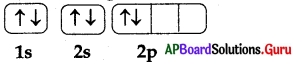A) Pauli’s exclusion principle
B) Hund’s rule
C) Aufbau principle
D) Heisenburg uncertainty principle
B) Hund’s rule

Question 78.
The principle is violated in the given information about Helium atom.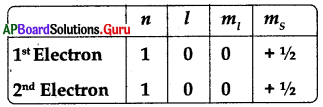A) Pauli’s exclusion principle
B) Hund’s rule
C) Aufbau principle
D) Heisenberg uncertainty principle
A) Pauli’s exclusion principle

Question 79.
According to Pauli’s exclusion principle the maximum number of electrons filled in an orbital is ………………
A) 1
B) 2
C) 3
D) 4
B) 2

Question 80.
The valence electron configuration of electron for the following quantum numbers.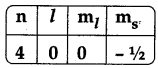A) 4s1
B) 4s2
C) 4p2
D) 4p2
B) 4s2

Question 81.
The valance electron configuration of electron for following quantum numbers.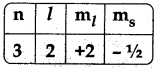A) 3d5
B) 3d6
C) 3d7
D) 3d10
D) 3d10

Question 82.
The following orbitals have n+l value 6
i) 5p
ii) 6s
iii) 4d
iv) 4f
A) only i
B) both i and ii
C) i, ii and iii
D) all of these
C) i, ii and iii

Question 83.
What are set of quantum numbers for electron configuration of element is 3d7?
A) n = 3, l = 2, ml = -1, ms = -1/2
B) n = 3, l =2, ml = -2, ms = -1/2
C) n = 3, l =2, ml= -1, ms = +1/2
D) n = 3, l = 2, ml= -2, ms = +1/2
A) n = 3, l = 2, ml = -1, ms = -1/2

Question 84.
The quantum number which says about size and energy of orbit is
A) n
B) l
C) ml
D) ms
A) n

Question 85.
Match the following:
a) Principal quantum number (n) — i) Orientation of orbital in space
b) Angular momentum quantum number (l) — Size and energy of orbit
c) Magnetic quantum number (ml) — iii) Shape of sub shell
d) Spin quantum number (ms )– Spin of electron
A) a→i, b→ii, c→iii, d→iv
B) a→ii, b→iii, c→i, d→iv
C) a→iv, b→iii, c→ii, d→i
D) a→iv, b→ii, c→iii, d→i
B) a→ii, b→iii, c→i, d→iv

Question 86.
If n = 3 and Z = 2 the energy level is represented as
A) 3s
B) 3p
C) 3d
D) 3f
C) 3dQuestion 87.
To which boundary surface diagram of d – orbital does indicate ?
A) dxy
B) dyz
C) dz2
D) dx2-y2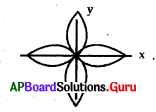D) dx2-y2

Question 88.
The number of possible orbitals in a sub shell with angular momentum quantum number (1) is
A) l +1
B) 2l + 1
C) 2 (21 + 1)
D) 0 ton-1
B) 2l + 1

Question 89.
The maximum number of electrons in n = 3 main energy level is ………………….
A) 8
B) 16
C) 18
D) 32
C) 18

Question 90.
Which of the following quantum number describes the orientation of orbital in space around the nucleus of atom?
A) Magnetic quantum number
B) Spin quantum number
C) Angular momentum quantum number
D) Principal quantum number
A) Magnetic quantum number

Question 91.
The correct electron configuration of Copper is……….
A) [Ar] 4s2 3d10
B) [Ar] 4s2 3d9
C) [Ar] 4s1 3d5
D) [Ar] 4s1 3d10
D) [Ar] 4s1 3d10

Question 92.
The correct electron configuration of Chromium is………..
A) [Ar] 4s1 3d5
B) [Ar] 4s2 3d5
C) [Ar] 4s1 3d10
D) [Ar] 4s2 3d10
A) [Ar] 4s1 3d5

Question 93.
The rule violated in electron configurations of Chromium and Copper is ………….
A) Aufbau
B) Hund
C) Pauli’s exclusion
D) Heisenberg uncertainty
A) Aufbau

Question 94.
Atoms having these orbitals are stable
A) half filled
B) completely filled
C) both A and B
D) none of these
C) both A and B

Question 95.
The maximum number of electrons accommodated in a sub shell with Azimuthal quantum number (angular momentum quantum number) l is ………………….
A) 2l + 1
B) 4l + 2
C) l(l+1)
D) 4l – 1
B) 4l + 2

Question 96.
The four quantum numbers for valance electron of Sodium atom are ………………
A) n = 1, l = 0, ml= 0, ms = +1/2
B) n = 2, l = 0, ml= 0, ms = +1/2
C) n = 3, l = 0, ml= 0, ms = +1/2
D) n = 3, l = 1, ml= 0, ms= +1/2
C) n = 3, l = 0, ml= 0, ms = +1/2

Question 97.
Degenerate orbitals have
A) same l value and same n value
B) different l value and same n value
C) same l value and different n value
D) same n+l value
A) same l value and same n valueQuestion 98.
When l = 3 the maximum number of electrons that can occupy in the corresponding sub shell is ……………
A) 2
B) 6
C) 10
D) 14
D) 14

Question 99.
The number of orbitals present in a given shell is …………
A) n
B) n2
C) 2n2
D) 2l + 1
B) n2

Question 100.
The orbital that is having least energy among 3p, 4s, 3d, 4p is ……………………..
A) 3p
B) 4s
C) 3d
D) 4p
A) 3p

Question 101.
Which of the following is the correct configuration of O2- …………….
A) 1s2 2s2 2p4
B) 1s2 2s2 2p6
C) 1s2 2s2 2p2
D) 1s2 2s2 2p5
B) 1s2 2s2 2p6

Question 102.
The impossible set of quantum numbers for any electron of an atom is ……………………
a) n = 1, l = 1, ml = 0, ms = +1/2
b) n = 2, l =2, ml = 1, ms = -1/2
c) n = 3, l = 2, ml = 1, ms = +1/2
d) n = 3, l = 0, ml = 0, ms = -1/2
A) a only
B) a, b only
C) c, d only
D) d only
B) a, b only

Question 103.
The orbitalwhich does not lie along the axis is………………
A) Px
B) d2 – y2
C) dxy
D) py
C) dxy

Question 104.
Which one of the following has the electronic configuration of 1s2 2s2 2p6?
A) Ne
B) Na+
C) F
D) All
D) All

Question 105.
Magnetic quantum number of the last electron of Sodium is ………………….
A) 3
B) 2
C) 1
D) 0